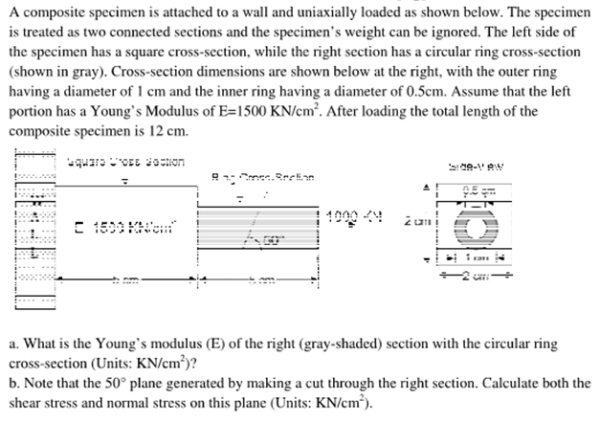A composite specimen is attached to a wall and uniaxially loaded as shown below. The speciment is treated as two connected sections and the specimen's weight can be ignored. The left side of the specimen has a square cross section, while the right section has a circular ring cross section (shown in gray). Cross section dimensions are shown below at the right, with the outer ring having a diameter of 1 cm and the inner ring having a diameter of 0.5 cm. Assume that the left portion has a Young's Modulus of E=1500 KN/cm^2. After loading, the total length of the composite specimen is 12 cm. a) What is the Young's modulus E of the right section with the circular ring cross section? b) Note the 50 degree plane generated by making a cut through the right section. Calculate both the shear stress and normal stress on this plane.A composite specimen is attached to a wall and uniaxially loaded as shown below. The speciment is treated as two connected sections and the specimen's weight can be ignored. The left side of the specimen has a square cross section, while the right section has a circular ring cross section (shown in gray). Cross section dimensions are shown below at the right, with the outer ring having a diameter of 1 cm and the inner ring having a diameter of 0.5 cm. Assume that the left portion has a Young's Modulus of E=1500 KN/cm^2. After loading, the total length of the composite specimen is 12 cm. a) What is the Young's modulus E of the right section with the circular ring cross section? b) Note the 50 degree plane generated by making a cut through the right section. Calculate both the shear stress and normal stress on this plane.

biomechanics page 1 biomechanics biomechanics biomechanics biomechanics biomechanics biomechanics biomechanics biomechanics page 2